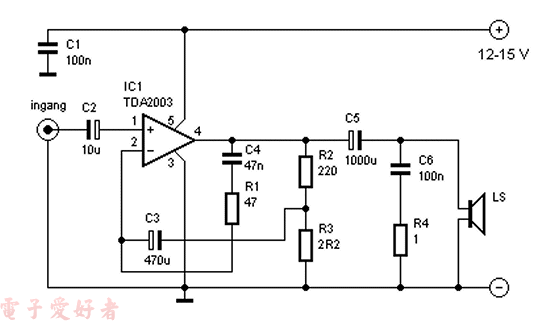# TDA2003十瓦音频放大器R1 = 47 Ω
R2 = 220 Ω
R3 = 2,2 Ω
R4 = 1 Ω
C1,C6 = 100 nF
C2 = 10 µF/16 V
C3 = 470 µF/16 V
C4 = 47 nF
C5 = 1000 µF/16 V
IC1 = TDA 2003